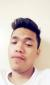# Engineering Mechanics -Dynamics

2 posts / 0 new
Kevin GarabilesEngineering Mechanics -Dynamics

( PASAGOT NAMAN PO ASSIGNMENT LANG PO HINDI KO TALAGA MASAGOT,, SALAMAT NG MARAMI.)An automobile is to travel a distance from A to B of 540m in exactly 4seconds. The auto accelerates and decelerates at 1.8 m/s^2, starting from rest A to rest B find velocity, maximum speed.

Jhun VertThe maximum speed will occur exactly midway between A and B because it accelerate and decelerate at the same rate at 1.8 m/s^2.

Formula to use:
$v^2 = {v_o}^2 + 2as$

Solve for v at s = 540/2

• Mathematics inside the configured delimiters is rendered by MathJax. The default math delimiters are $$...$$ and $...$ for displayed mathematics, and $...$ and $...$ for in-line mathematics.